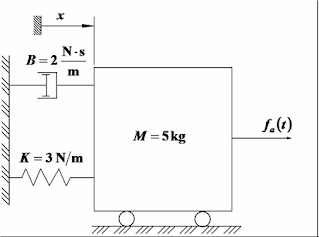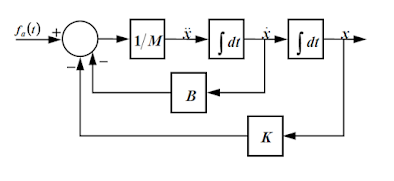## Welcome to the World of Modelling and Simulation

### What is Modelling?

This blog is all about system dynamics modelling and simulation applied in the engineering field, especially mechanical, electrical, and ...### A Short MATLAB Script for Spectrum Analysis or FFT

The following codes may be used for spectrum analysis for any vibration problems. The program is capable of isolating fundamental peaks from a noisy signal smoothly. It uses the MATLAB built-in Fast Fourier Transform (FFT) algorithm.

Codes for Spectrum Analysis (FFT):

NS=EFFORTS(:,1);                 % Data to Analyze
L=length(NS);
Fs=L;                            % Sample Rate
NFFT = 2^nextpow2(L);
F = Fs/2*linspace(0,1,NFFT/2+1);
win=hamming(L,'periodic');
Ywin=win.*NS;
X = fft(Ywin,NFFT)/L;            % MATLAB built-in FFT Algorithm
Xf=abs(X(1:NFFT/2+1));
Xf=Xf/max(Xf);                   % Normalizing Magnitude

plot(F,Xf)

### Observation of a Typical Open Loop Dynamic Responses of Second Order Systems

Dynamic Responses
In vibration and control, we always deal with the following three types of the dynamic responses from a system due to the step input to that system. These responses are: over damped, critically damped, and under damped signals.

• Overdamped - where the damping ratio is higher than the expected or optimum. As the name suggests, 'damped' means to stabilize or settle down a signal, and over-damped is in the same perspective that the signal is sized or filtered overly to reach a desired value. In the following response curves, the yellow colored signal represents an overdamped response.
• Underdamped - where the damping ratio is below the desired limit. Again, from its title, 'under' defines that the signal is not sufficiently filtered or processed to reach the optimum or desired level. The light blue colored signal in the following response curve shows the underdamped signal response. We see that the signal has couple of overshoots and undershoots until it settles down to the desired value.
• Critically Damped - This is the response that we desire to achieve, which means that the system response to the step input should have a shape that is shown by the pink colored signal in the following response curve.

The following block diagram represents the generation of three types of responses using three SIMULINK transfer function blocks.

Response Curves

#OverDamped   #UnderDamped   #CriticallyDamped   #DynamicResponse

### Modelling a Basic Second Order System in SIMULINK

Problem: Consider the mechanical system shown in the figure below. The system is at rest for t < 0. At t = 0 an impulseforce of 8 N is applied to the mass. The impulse lasts 0.06 seconds. The displacement x is measured from the equilibrium position just before the mass is hit by the impulse force. Find the mathematical model of the system, draw a block diagram to represent it, and plot the displacement and velocity of the mass during the first 25 seconds.Figure 1: Diagram of the System.

Solution: The mathematical model is given by the following equation:The block diagram for the system is given below:Figure 2: Block Diagram.Figure 3: Simulink Block Diagram.

The output variables (i.e., displacement and velocity of the mass) displayed by the scope is shown in the figure below.

After examining the SIMULINK model, you can see that blocks from the following libraries have been used:
• Sources library: the Pulse Generator block (the Clock block was not used because it was not requested to send any variable to the MATLAB Workspace)
• Sinks library: The Scope block
• Math Operations library: The Sum and Gain blocks
• Continuous library: The Integrator block
• Signal Routing library: The Mux blockFigure 4: Response Curve.

You should have noticed that two of the gain blocks (damper and spring) have been rotated 180 degrees with respect to its default orientation. This has been done by right clicking on the corresponding block and then selecting flip block from the format menu. In this case the applied load was modeled using the Pulse Generator block. The dialog box containing the parameters for this block is shown below.

### An Iterative Approach to Solve a System of Equations

In this tutorial, we are going to solve the following two algebraic equations iteratively.

X₁ = X₂ - 1
X₂ = 2.5 X₁ - 0.5

Using fixed point iterative method and taking initial assumptions according to the question as, X₁ = X₂ = 0, the solutions do not converge with this present arrangement of equations.
However, if we rearrange these equations in the following forms, the solutions indeed converge.

X2 = X1 + 1
X₁ = X₂ / 2.5 + 0.2

A MATLAB program has been developed to solve the modified equations iteratively by creating a user defined function called "iterative". This function may be used to solve other equations iteratively which is the essence of the user defined function. The previous forms of equations from the question are also fed into the program to see the solutions, but the program indicates divergence.
The following MATLAB program written in “m-file” subsequently enlightens essential commands in the program by additional notes.

MATLAB Codes:
% Definition of a function "iterative" to solve equations iteratively

function [x1, x2, ea1, ea2] = iterative(X1, X2, etol1, etol2)

% Input X1=0, X2=0, etol1, etol2=Tolerance definition for errors
% Output x1, x2=Solutions of equations, ea1, ea2= Calculated errors in loop

% Program Initialization

solution1 = 0;
solution2 = 0;

% Iterative Calculations

while (1)

solutionprevious1 = solution1;

solutionprevious2 = solution2;

solution2 = X1+1;             % Problem equation 1

solution1 = (X2/2.5)+0.2;     % Problem equation 2

X1=solution1;

X2=solution2;

if solution1~=0 && solution2~=0

% Approximate percent relative errors

ea1=abs((solution1 - solutionprevious1)/solution1)*100;

ea2=abs((solution2 - solutionprevious2)/solution2)*100;

end

if ea1<=etol1 && ea2<=etol2,  % Conditions to meet specified error tolerances

break,

end

x1 = solution1;

x2 = solution2;

end

% Display of output parameters

disp(x1);

disp(x2);

disp(ea1);

disp(ea2);

end

Outputs:
>> iterative (0,0,1e-5,1e-5)      % Command input at the MATLAB command prompt
1.0000
2.0000
3.4360e-06
8.5899e-06

Therefore, the solutions obtained from the program are X₁ = 1 and X₂ = 2. And, the approximate percent relative errors for finding X₁, X₂ are 3.4360e-06 and 8.5899e-06 respectively which also satisfy the condition mentioned in the program.

### Newton-Raphson Method Codes for MATLAB

Newton-Raphson method is implemented here to determine the roots of a function. This algorithm is coded in MATLAB m-file. There are three files: func.m, dfunc.m and newtonraphson.m. The func.m defines the function, dfunc.m defines the derivative of the function and newtonraphson.m applies the Newton-Raphson method to determine the roots of a function.

Evaluating the root of sin(x):

func.m file
% defines the function as its roots to be determined

function [solution]=func(x)
solution=sin(x);

dfunc.m file
% defines the derivative of the function

function [dsolution]=dfunc(x)
dsolution=cos(x);

newtonraphson.m file
% applies Newton Raphson algorithm to determine the roots of a function

close all;
clear all;
x = input('Starting guess = ');
tolerance = 1e-8;
iterations = 0;
while (iterations<100) && (abs(func(x))>tolerance)
x = x-(func(x)/dfunc(x));
iterations = iterations + 1;
end
if iterations==100
disp('No root found')
else
disp(x)
end

Output:

Starting guess = 1

2.9236e-13

Starting guess = 0.1

1.2495e-11

Starting guess = 0.01

1.2335e-20

Evaluating the root of x-2*sin(xˆ2):

func.m file
% defines the function as it's roots to be determined

function [solution]=func(x)
solution = x-(2*sin(x.^2));

dfunc.m file
% defines the derivative of the function

function [dsolution]=dfunc(x)
dsolution=1-(4*x*cos(x));

Output:

Starting guess = 0.1

-1.0489e-10

Starting guess = 0.3

-2.4565e-10

Starting guess = 1

0.5055

Evaluating the root of x^2-5:

func.m file
% defines the function as it's roots to be determined

function [solution]=func(x)
solution = x^2-5;

dfunc.m file
% defines the derivative of the function

function [dsolution]=dfunc(x)
dsolution = (2*x);

Output:

Starting guess = 2

2.2361

Starting guess = 3

2.2361

Starting guess = 0.01

2.2361

#NewtonRaphson

### Exploring Some MATLAB Basic Commands

In this post, we will explore some of the basic built-in MATLAB commands.

Generation of Random Numbers (“rand” command):

The thousand random numbers are generated by the following MATLAB command. In the following table 1, 100 of the 1000 generated random numbers are shown.

>> a=rand(1000,1)Random Number Sorting:

>> b=sort(a)
The sorted numbers (100 out of the 1000) are shown in table 2.>> c=mean(b)
c = 0.5089

>> d=median(b)
d = 0.5162

>> e=mode(b)
e = 3.4146e-04

>> f=var(b)
f = 0.0818

>> g=std(b)
g = 0.2860

>> h=bar(b)
h = 174.0016

The standard uniform distribution is the simplest distribution for continuous random variable.  In this case the probability of occurrence of each event is same and when the probability of occurrence is plotted against the numbers, it gives a horizontal line.  In standard uniform distribution, the lowest boundary is 0 and the highest boundary is 1. So, the area under the horizontal line always gives 1. The distribution is rectangular in shape which is defined by maximum and minimum values.Figure 1: Showing the bar plot of the uniformly distributed random numbers.

Generation of Random Numbers (“randn” command):

The thousand random numbers are generated by the following MATLAB command. In the following table 3, 100 of the 1000 generated random numbers are shown.

>>a=randn(1000,1)

>>b=sort(a)

>> c=mean(b)
c =    0.0512

>> d=median(b)
d =   0.0507

>> e=mode(b)
e = -3.4915

>> f=var(b)
f = 1.0212

>> g=std(b)
g = 1.0105

>> h=bar(b)
h = 174.0031

The standard normal distribution is probably the most useful tool in solving many problems. It is a bell shaped curve and has a peak value at its center. This distribution is symmetric about the mean and also asymptotic (the curve gets closer and closer to the x-axis however never touches the axis). The total area under the curve is 1. The position of a normal distribution is determined by the mean and standard deviation as well.Figure 2: Showing the bar plot of the normally distributed random numbers